Main MRPT website > C++ reference for MRPT 1.5.6
mrpt::math::CMatrixD Class Reference

Detailed Description

This class is a "CSerializable" wrapper for "CMatrixTemplateNumeric<double>".

Note
For a complete introduction to Matrices and vectors in MRPT, see: http://www.mrpt.org/Matrices_vectors_arrays_and_Linear_Algebra_MRPT_and_Eigen_classes

Definition at line 30 of file CMatrixD.h.

#include <mrpt/math/CMatrixD.h>

Inheritance diagram for mrpt::math::CMatrixD: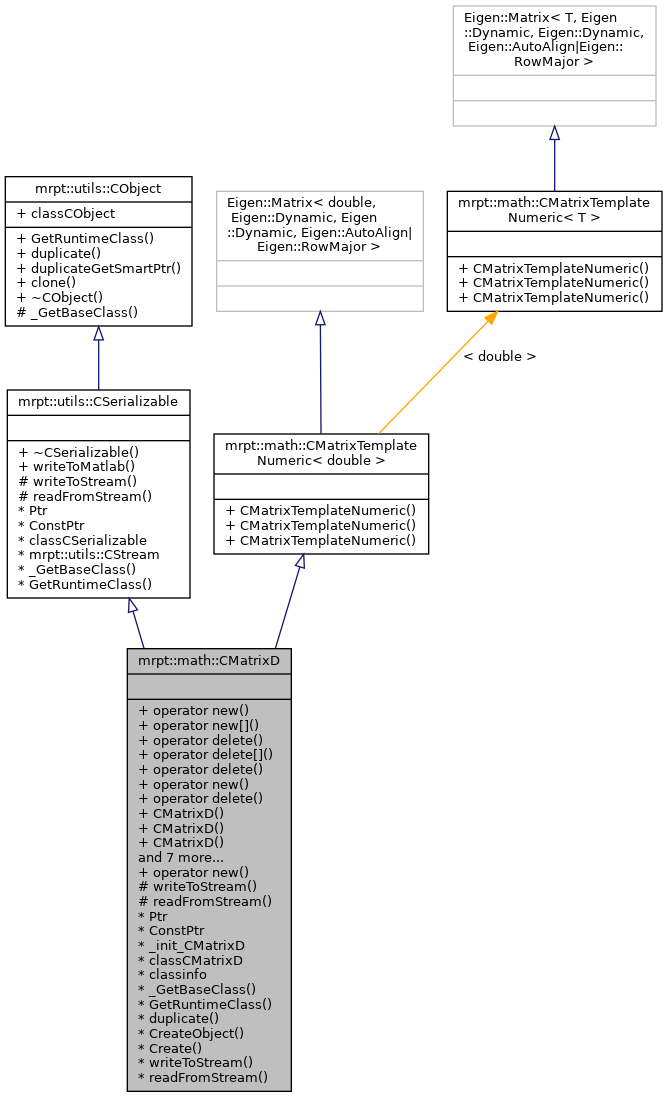Public Types

typedef Eigen::Matrix< double,
Eigen::Dynamic, Eigen::Dynamic,
Eigen::AutoAlign|Eigen::RowMajor >
Base

typedef CMatrixTemplateNumeric
< double >
mrpt_autotype

Public Member Functions

voidoperator new (size_t size)

voidoperator new[] (size_t size)

void operator delete (void *ptr) throw ()

void operator delete[] (void *ptr) throw ()

void operator delete (void *memory, void *ptr) throw ()

voidoperator new (size_t size, const std::nothrow_t &) throw ()

void operator delete (void *ptr, const std::nothrow_t &) throw ()

CMatrixD ()
Constructor. More...

CMatrixD (size_t row, size_t col)
Constructor. More...

CMatrixD (const CMatrixTemplateNumeric< double > &m)
Copy constructor. More...

CMatrixD (const CMatrixFloat &m)
Copy constructor. More...

template<typename OtherDerived >
CMatrixDoperator= (const Eigen::MatrixBase< OtherDerived > &other)

template<typename OtherDerived >
CMatrixD (const Eigen::MatrixBase< OtherDerived > &other)

CMatrixD (const TPose2D &p)
Constructor from a TPose2D, which generates a 3x1 matrix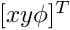. More...

CMatrixD (const TPose3D &p)
Constructor from a TPose3D, which generates a 6x1 matrix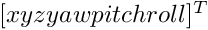. More...

CMatrixD (const TPoint2D &p)
Constructor from a TPoint2D, which generates a 2x1 matrix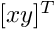. More...

CMatrixD (const TPoint3D &p)
Constructor from a TPoint3D, which generates a 3x1 matrix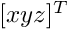. More...

virtual mxArraywriteToMatlab () const
Introduces a pure virtual method responsible for writing to a mxArray Matlab object, typically a MATLAB struct whose contents are documented in each derived class. More...

mrpt::utils::CObjectPtr duplicateGetSmartPtr () const
Returns a copy of the object, indepently of its class, as a smart pointer (the newly created object will exist as long as any copy of this smart pointer). More...

CObjectclone () const
Cloning interface for smart pointers. More...

Static Public Member Functions

static voidoperator new (size_t size, void *ptr)

Static Public Attributes

static const
mrpt::utils::TRuntimeClassId
classCObject

RTTI stuff
static const
mrpt::utils::TRuntimeClassId
classCSerializable

Protected Member Functions

CSerializable virtual methods
void writeToStream (mrpt::utils::CStream &out, int *getVersion) const
Introduces a pure virtual method responsible for writing to a CStream. More...

void readFromStream (mrpt::utils::CStream &in, int version)
Introduces a pure virtual method responsible for loading from a CStream This can not be used directly be users, instead use "stream >> object;" for reading it from a stream or "stream >> object_ptr;" if the class is unknown apriori. More...

RTTI stuff

typedef CMatrixDPtr Ptr

typedef CMatrixDPtr ConstPtr

static mrpt::utils::CLASSINIT _init_CMatrixD

static mrpt::utils::TRuntimeClassId classCMatrixD

static const
mrpt::utils::TRuntimeClassId
classinfo

static const
mrpt::utils::TRuntimeClassId
_GetBaseClass ()

virtual const
mrpt::utils::TRuntimeClassId
GetRuntimeClass () const
Returns information about the class of an object in runtime. More...

virtual mrpt::utils::CObjectduplicate () const
Returns a copy of the object, indepently of its class. More...

static mrpt::utils::CObjectCreateObject ()

static CMatrixDPtr Create ()

Member Typedef Documentation

 typedef Eigen::Matrix mrpt::math::CMatrixTemplateNumeric< double >::Base
inherited

Definition at line 42 of file CMatrixTemplateNumeric.h.

 typedef CMatrixDPtr mrpt::math::CMatrixD::ConstPtr

Definition at line 33 of file CMatrixD.h.

 typedef CMatrixTemplateNumeric mrpt::math::CMatrixTemplateNumeric< double >::mrpt_autotype
inherited

Definition at line 43 of file CMatrixTemplateNumeric.h.

 typedef CMatrixDPtr mrpt::math::CMatrixD::Ptr

A typedef for the associated smart pointer

Definition at line 33 of file CMatrixD.h.

Constructor & Destructor Documentation

 mrpt::math::CMatrixD::CMatrixD ( )
inline

Constructor.

Definition at line 36 of file CMatrixD.h.

 mrpt::math::CMatrixD::CMatrixD ( size_t row, size_t col )
inline

Constructor.

Definition at line 40 of file CMatrixD.h.

 mrpt::math::CMatrixD::CMatrixD ( const CMatrixTemplateNumeric< double > & m )
inline

Copy constructor.

Definition at line 44 of file CMatrixD.h.

 mrpt::math::CMatrixD::CMatrixD ( const CMatrixFloat & m )
inline

Copy constructor.

Definition at line 48 of file CMatrixD.h.

template<typename OtherDerived >
 mrpt::math::CMatrixD::CMatrixD ( const Eigen::MatrixBase< OtherDerived > & other )
inline

Constructor from any other Eigen class

Definition at line 60 of file CMatrixD.h.

 CMatrixD::CMatrixD ( const TPose2D & p )
explicit

Constructor from a TPose2D, which generates a 3x1 matrix.

Definition at line 24 of file CMatrixD.cpp.

 CMatrixD::CMatrixD ( const TPose3D & p )
explicit

Constructor from a TPose3D, which generates a 6x1 matrix.

Definition at line 26 of file CMatrixD.cpp.

 CMatrixD::CMatrixD ( const TPoint2D & p )
explicit

Constructor from a TPoint2D, which generates a 2x1 matrix.

Definition at line 28 of file CMatrixD.cpp.

 CMatrixD::CMatrixD ( const TPoint3D & p )
explicit

Constructor from a TPoint3D, which generates a 3x1 matrix.

Definition at line 30 of file CMatrixD.cpp.

Member Function Documentation

 static const mrpt::utils::TRuntimeClassId* mrpt::math::CMatrixD::_GetBaseClass ( )
staticprotected
 CObject* mrpt::utils::CObject::clone ( ) const
inlineinherited

Cloning interface for smart pointers.

Definition at line 143 of file CObject.h.

 static CMatrixDPtr mrpt::math::CMatrixD::Create ( )
static
 static mrpt::utils::CObject* mrpt::math::CMatrixD::CreateObject ( )
static
 virtual mrpt::utils::CObject* mrpt::math::CMatrixD::duplicate ( ) const
virtual

Returns a copy of the object, indepently of its class.

Implements mrpt::utils::CObject.

 mrpt::utils::CObjectPtr mrpt::utils::CObject::duplicateGetSmartPtr ( ) const
inlineinherited

Returns a copy of the object, indepently of its class, as a smart pointer (the newly created object will exist as long as any copy of this smart pointer).

Definition at line 140 of file CObject.h.

 virtual const mrpt::utils::TRuntimeClassId* mrpt::math::CMatrixD::GetRuntimeClass ( ) const
virtual

Returns information about the class of an object in runtime.

Reimplemented from mrpt::utils::CSerializable.

 void mrpt::math::CMatrixD::operator delete ( void * ptr ) throw ( )
inline

Definition at line 33 of file CMatrixD.h.

 void mrpt::math::CMatrixD::operator delete ( void * memory, void * ptr ) throw ( )
inline

Definition at line 33 of file CMatrixD.h.

 void mrpt::math::CMatrixD::operator delete ( void * ptr, const std::nothrow_t & ) throw ( )
inline

Definition at line 33 of file CMatrixD.h.

 void mrpt::math::CMatrixD::operator delete[] ( void * ptr ) throw ( )
inline

Definition at line 33 of file CMatrixD.h.

 static void* mrpt::math::CMatrixD::operator new ( size_t size, void * ptr )
inlinestatic

Definition at line 33 of file CMatrixD.h.

 void* mrpt::math::CMatrixD::operator new ( size_t size )
inline

Definition at line 33 of file CMatrixD.h.

 void* mrpt::math::CMatrixD::operator new ( size_t size, const std::nothrow_t & ) throw ( )
inline

Definition at line 33 of file CMatrixD.h.

 void* mrpt::math::CMatrixD::operator new[] ( size_t size )
inline

Definition at line 33 of file CMatrixD.h.

template<typename OtherDerived >
 CMatrixD& mrpt::math::CMatrixD::operator= ( const Eigen::MatrixBase< OtherDerived > & other )
inline

Assignment operator from any other Eigen class

Definition at line 54 of file CMatrixD.h.

 void CMatrixD::readFromStream ( mrpt::utils::CStream & in, int version )
protectedvirtual

Introduces a pure virtual method responsible for loading from a CStream This can not be used directly be users, instead use "stream >> object;" for reading it from a stream or "stream >> object_ptr;" if the class is unknown apriori.

Parameters
 in The input binary stream where the object data must read from. version The version of the object stored in the stream: use this version number in your code to know how to read the incoming data.
Exceptions
 std::exception On any error, see CStream::ReadBuffer
CStream

Implements mrpt::utils::CSerializable.

Definition at line 55 of file CMatrixD.cpp.

 virtual mxArray* mrpt::utils::CSerializable::writeToMatlab ( ) const
inlinevirtualinherited

Introduces a pure virtual method responsible for writing to a mxArray Matlab object, typically a MATLAB struct whose contents are documented in each derived class.

Returns
A new mxArray (caller is responsible of memory freeing) or NULL is class does not support conversion to MATLAB.

Definition at line 79 of file CSerializable.h.

 void CMatrixD::writeToStream ( mrpt::utils::CStream & out, int * getVersion ) const
protectedvirtual

Introduces a pure virtual method responsible for writing to a CStream.

This can not be used directly be users, instead use "stream << object;" for writing it to a stream.

Parameters
 out The output binary stream where object must be dumped. getVersion If NULL, the object must be dumped. If not, only the version of the object dump must be returned in this pointer. This enables the versioning of objects dumping and backward compatibility with previously stored data.
Exceptions
 std::exception On any error, see CStream::WriteBuffer
CStream

Implements mrpt::utils::CSerializable.

Definition at line 36 of file CMatrixD.cpp.

Member Data Documentation

 mrpt::utils::CLASSINIT mrpt::math::CMatrixD::_init_CMatrixD
staticprotected

Definition at line 33 of file CMatrixD.h.

 mrpt::utils::TRuntimeClassId mrpt::math::CMatrixD::classCMatrixD
static

Definition at line 33 of file CMatrixD.h.

 const mrpt::utils::TRuntimeClassId mrpt::utils::CObject::classCObject
staticinherited

Definition at line 128 of file CObject.h.

 const mrpt::utils::TRuntimeClassId mrpt::utils::CSerializable::classCSerializable
staticinherited

Definition at line 42 of file CSerializable.h.

 const mrpt::utils::TRuntimeClassId* mrpt::math::CMatrixD::classinfo
static

Definition at line 33 of file CMatrixD.h.

 Page generated by Doxygen 1.8.6 for MRPT 1.5.6 Git: 4c65e84 Tue Apr 24 08:18:17 2018 +0200 at mar abr 24 08:26:17 CEST 2018